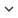ENGArticle
Article 365
Author/s
• Nagy D., Indalecio G., Garcia-Loureiro A., Elmessary M., Kalna K., Seoane N.
DOI
Source
• IEEE Transactions on Electron Devices, 2017 - Q1
Research Areas

# Metal Grain Granularity Study on a Gate-All-Around Nanowire FET

IEEE The impact of the metal grain granularity (MGG) variations on subthreshold and ON-current of a 22-nm gate length Si gate-all-around (GAA) nanowire (NW) field-effect transistor (FET) is analyzed by assessing five figures-of-merit: threshold voltage (VT), off-current (IOFF), subthreshold slope (SS) and drain-induced-barrier-lowering at low (VD = 50 mV) and high (VD = 1.0 V) drain biases, and ON-current variability at the high drain bias. We assume a TiN metal gate with four grain sizes (GS): 10, 7, 5, and 3 nm. The simulations carried out employ: 1) a 3-D finite element (FE) drift-diffusion (DD) simulator with density-gradient (DG) quantum corrections (QC) in the subthreshold region and 2) a 3-D FE Monte Carlo (MC) simulation toolbox with Schr & #x00F6;dinger equation QC for the ON-current. We have found that the & #x03C3;VT due to the MGG variability is 10 & #x0025; higher in the GAA-NW FET (GS of 10 nm) than in a 20-nm gate FinFET. For a GS of 3 nm, the variability becomes comparable between the GAA-NW FET and the FinFET. Comparison against the line-edge-roughness (LER) variability shows that the MGG (GS of 10 nm) affects & #x03C3;VT 92 & #x0025; more than the LER (root-mean-square height of 0.85 nm and correlation length of 20 nm). Finally, the ON-current of the FinFET (GS of 10 nm, VD = 0.9 V) has a 43 & #x0025; higher & #x03C3;ION than that of the GAA-NW (GS of 10 nm, VD = 1.0 V) making the GAA-NW FETs a much better solution for sub-10-nm technology nodes.
Keywords: Density gradient (DG) quantum corrections (QC), drift-diffusion (DD), FinFETs, gate work function variability, Iron, line edge roughness (LER), Logic gates, metal grain granularity (MGG), Monte Carlo simulations (MC), Nanoscale devices, Schr&amp;#x00